## Introduction

Quantum computing, as conceived by Feynman1, has the potential to revolutionize computing for certain classes of problems with exponential scaling in the physical and social sciences and engineering2,3,4,5,6,7,8,9,10. Central to the quantum computing paradigm is the quantum process of entanglement by which a pure-state quantum system develops a probability distribution over multiple classical outcomes. Entanglement allows us to process and store exponentially more information than a classical computer. This potential capability and its advantages, however, come with a significant sensitivity to noise6,11,12,13 that introduces errors that degrade performance, especially on current-to-near-term quantum computers. Significant advances have been made in the past decade in error correction and mitigation14,15,16,17,18,19, but further advances are needed to not only understand noise but also control noise for its mitigation or exploitation.

In quantum mechanics, a closed system which is in a stationary state will remain in that state for all time. If, however, the closed quantum system is opened to an environment, also referred to as a bath, then the system becomes an open-quantum system, and a stationary state of that system will potentially become time-dependent and non-stationary11. The precise time dependence of the open quantum system depends upon the nature of the bath. If the relaxation of the bath is fast relative to the dynamics of the system, then the quantum dynamics is purely dissipative and described as Markovian, but if the dynamics of the bath and system are on the same timescale, then the dynamics causes energy to be exchanged both to and from the bath and is described as non-Markovian11,13,20. In non-Markovian dynamics the more complex interaction between the system and bath causes the system to develop a memory of its state as a function of time.

We can broadly characterize noise or the effects of noise through spectroscopic or tomographic techniques. In the context of quantum computing, characterization of the bath, which is commonly assumed to be Markovian, gives a basic assessment of the qubit and gate performance. While quantum process tomographic techniques can be used21,22,23,24, they are costly and potentially unreliable for realistic measurement, and other alternatives such as randomized benchmarking or quantum gate set tomography have emerged as more robust tools25,26,27. Non-Markovian behavior is present in a variety of systems, but is generally more challenging to characterize28,29. Efficient characterization of an underlying interaction is also possible for one or two-qubit systems30,31. In the frame of a simulated quantum system, however, we are not usually concerned with the characterization of the device, but the bath in relation to the simulated system.

In this paper, we simulate stationary states on a quantum computer to obtain a unique spectroscopic fingerprint of the computer’s noise. If a quantum system in a stationary state is simulated on an ideal quantum computer, the quantum system will remain in that stationary state for all time. However, if the same system is simulated on a noisy intermediate-scale quantum (NISQ) computer, the noise causes the simulated state to become non-stationary. The resulting time dependence in the frame of the simulation provides us with a frequency profile of the noise as it is experienced by the simulated stationary quantum state. Knowledge of the bath in the frame of the simulated system can potentially be utilized in the simulation of other noisy quantum systems or the design of better algorithms for quantum simulations32,33. Computations are performed on multiple superconducting-qubit IBM quantum computers. We find that each quantum computer has a unique spectroscopic signature for a given simulation of stationary states. The noise generates an effective bath that exhibits both colored noise and non-Markovian behavior11,13,20. Characterization of the bath provides an application-oriented assessment of the fidelity of the quantum device. Our results provide a direction for noise mitigation but also suggest how to use noise for quantum simulations of open systems8,34,35,36,37,38,39,40,41.

## Results

### Stationary-state evolution with noise

Time evolution of a stationary state prepared on a noisy quantum computer can be described by the equation of motion of the density matrix D according to the Nakajima–Zwanzig integro-differential equation11,13,42

$$\frac{dD}{dt}=-\frac{i}{\hslash }[\hat{H},D]+\int\nolimits_{0}^{t}{{{{{{{\mathcal{K}}}}}}}}(t,\tau )D(t,\tau )d\tau ,$$
(1)

where $$\hat{H}$$ is the Hamiltonian operator of the stationary state and $${{{{{{{\mathcal{K}}}}}}}}(t,\tau )$$ is the memory kernel representing the quantum computer’s noise. The memory kernel as represented here also includes the contribution of memoryless (i.e., Markovian) noise effects, which can be represented by a Dirac delta function at t in the kernel. In the limit that the noise on the quantum computer vanishes, the memory kernel vanishes and the equation simplifies to the quantum Liouville (von Neumann) equation. If the initial state is a stationary state of $$\hat{H}$$, all of the non-trivial time dependence results from the noise. Normal noise spectroscopy or characterization of a system could be performed by using a number of techniques, such as with Rabi spectroscopy, swap spectroscopy, or with a tunable system43,44,45,46,47,48,49,50,51. Here, to probe the frequency dependence of the noise, we consider the family of scaled Hamiltonians $$\hat{H}=\hslash \omega \hat{O}$$ where $$\hat{O}$$ is a dimensionless operator. By changing ω, we can control the energy difference between the ground and the first excited state of the simulated system. The time-dependent response of the system to different values of ω provides us with spectral information about the noise on the quantum computer. From one perspective we are attempting to simulate the solution of the quantum Liouville equation on the quantum computer with the memory kernel set to zero. Consequently, all deviations from the closed-system evolution are originating from the noise of the given quantum computer which creates without our direction an effective memory kernel for the time evolution (including memoryless effects).

### Single-qubit Hamiltonians

We begin with a single-qubit system with the Hamiltonian matrix H(ω) = ωσz where we use atomic units with  = 1, and σz is the Pauli-Z matrix,

$${\sigma }_{z}=\left(\begin{array}{rc}1&0\\ 0&-1\end{array}\right).$$
(2)

We prepare the system in the excited state $$|\psi\rangle =\left|1\right\rangle$$ and evolve the system according to $$\exp [-iH\tau ]={R}_{z}(2\omega \tau )$$, using repeated single-qubit gates with the results shown in Fig. 1. The time step is arbitrary to the extent that it can be rescaled with the strength of the Hamiltonian (in this case, ω), and hence, we set $$\tau =\frac{1}{3}$$, which serves to highlight system-bath interactions when the frequency ω [0, 1]. If we allowed the system to relax without applying any gates, this would essentially be a T1 experiment (where we could use the physical gate times), measuring the relaxation time for an excited state. However, the noise sources here, represented by the non-vanishing memory kernel, generate non-Markovian behavior. In Fig. 1, the non-Markovian behavior can be seen from the oscillations in the population of the ground state, which reveal a memory dependence beyond the pure decay of Markovian dynamics. Furthermore, the oscillations are more pronounced at lower frequencies, indicating a bath with colored noise.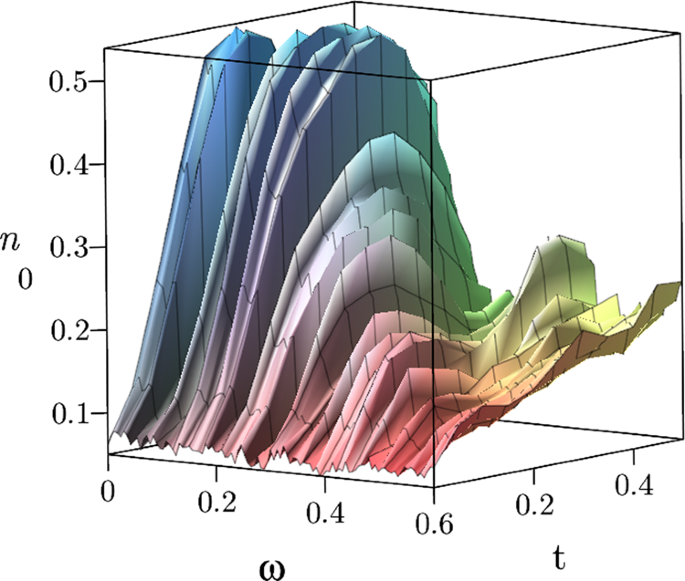Fig. 1: Frequency scan of simulated time evolution for a single qubit.

The spectral density with respect to a quantum noise source A can be characterized as49:

$${S}_{A}(\omega ) =\int\nolimits_{-\infty }^{\infty }d\tau \exp [i\omega \tau ]\mathop{\sum}\limits_{\alpha \beta }{\rho }_{\alpha \alpha }\langle \alpha | A(\tau )| \beta \rangle \langle \beta | A(0)| \alpha \rangle \\ =2\pi \mathop{\sum}\limits_{\alpha ,\beta }{\rho }_{\alpha \alpha }| {A}_{\alpha \beta }{| }^{2}\delta ({\epsilon }_{\beta }-{\epsilon }_{\alpha }-\omega ),$$
(3)

where α and β are energy eigenstates of H and ρ represents the density matrix. The spectral density can be related to the rate of population change through first-order perturbation theory with the well-known Fermi’s golden rule12,49,52. Figure 2 is constructed from Fig. 1, showing the rate of change in the initial part of the time evolution as a function of frequency, which provides a rough spectrum of the noise on the quantum computer from the perspective of the simulated system. Note that for frequencies above 0.6 (relative to a value of τ = 1/3), the noise profile shows low-intensity signals, likely from thermal noise. The appropriate resolution is difficult to determine, and the wells are not stochastic, as a purely stochastic phenomenon would not be continuous along the time series since each time in the series is sampled independently.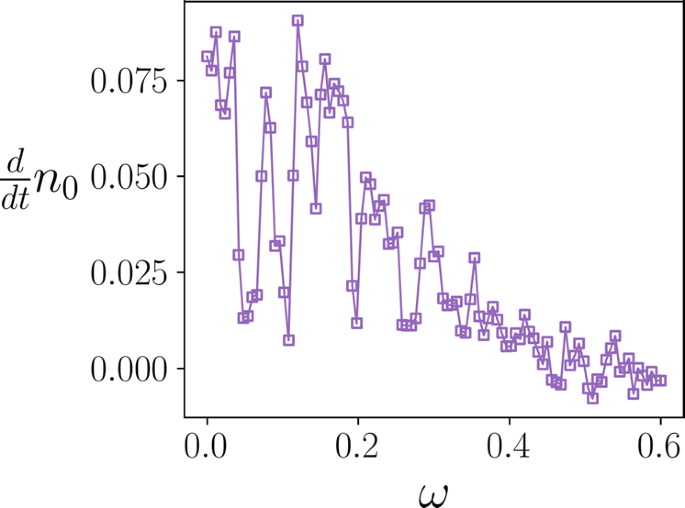Fig. 2: Rate of population change with respect to simulated time for different frequencies with a single-qubit system.

The significant portion of the noise can be ascribed to a transverse noise source (described by the Pauli-X or -Y matrices) which allows for transitions between the ground and excited states. The quantum devices we studied utilize a sequence of elementary gates denoted as U1, U2, and U3 gates. In particular, these gates consist of alternating frame changes (Rz gates) and rotations (X90 rotation, Rx gates), where Uk contains k frame changes and k − 1 transverse rotations (in the current iteration of IBM systems, these gates are represented with a series of intertwined Rz and $$\sqrt{X}$$ gates, which can also be used in a similar manner to represent generic single-qubit unitary transformations). If only the U1 gate is used to model $$\exp [-i\omega {\sigma }_{z}\tau ]$$, then a pulse is not applied, and the gate applications correspond to a standard T1 experiment, measuring the relaxation of the excited state. However, here we specifically use U3 gates, which are generic single-qubit rotations, and which alternate between transverse and longitudinal rotations. In fact, one way to model similar behavior in the qubit system is to apply a constant $$\exp [i\theta {\sigma }_{x}]$$, where θ is taken to be a small angle, following every gate application. However, this does not account for the troughs seen within the range from 0.03 to 0.3. Whether or not the noise channels are a result of an improper calibration of the X90 gate resulting in a systematic overrotation, or are part of a completely different noise source, becomes irrelevant for the noisy system in that the quantum system could be equivalently described from either perspective45,53. For a user or algorithm that does not control the qubit and gate level of the simulation, we posit that the effects of the two are the same, and the quantum system could be viewed as experiencing the same.

In addition to using different devices, we also can highlight system-specific responses on a single device by choosing different qubits to simulate our system. We demonstrate this in Fig. 3. In Fig. 3a, b, c, d, it appears that only one or two frequencies in the simulated evolution show a frequency-dependent response, which in some instances appears to be Markovian. However, for others, like in Fig. 3e, f, g, h we see strong non-Markovian behavior at numerous frequencies. The highest frequencies in each case appear to exhibit simple exponential decay, and so can be taken as an indicator of the simulated relaxation time. Note that a strict comparison cannot be made between the simulated relaxation time and the qubit performance characteristics. For single qubit with high-fidelity gates, we might expect the T1 time to mirror the simulated relaxation rate (see Supplementary Note I for an explicit comparison). In practice, however, gate errors obfuscate the comparison by contributing to the simulated relaxation rates. Also, the evolution by gate applications introduces energy into the system, which causes the system to relax into a mixed ensemble state rather than the ground state.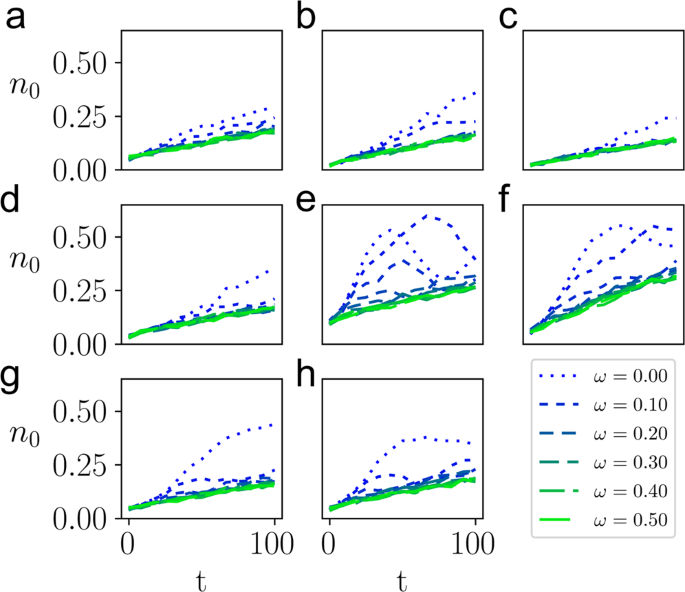Fig. 3: Demonstration of simulated time evolution for different devices and qubits.

We can also observe how the quantum state vector moves in the Bloch sphere at different frequencies. For frequencies in the high region of the spectral density and not allowing for coupling between the bath and system, a slow precession around the axis corresponding to H is observed. However, for frequencies which couple to the bath, the system can be strongly pulled around the Bloch sphere resulting in various trajectories through the state space. We model some of the trajectories in Fig. 4, and instances with other Hamiltonians are included in Supplementary Note I.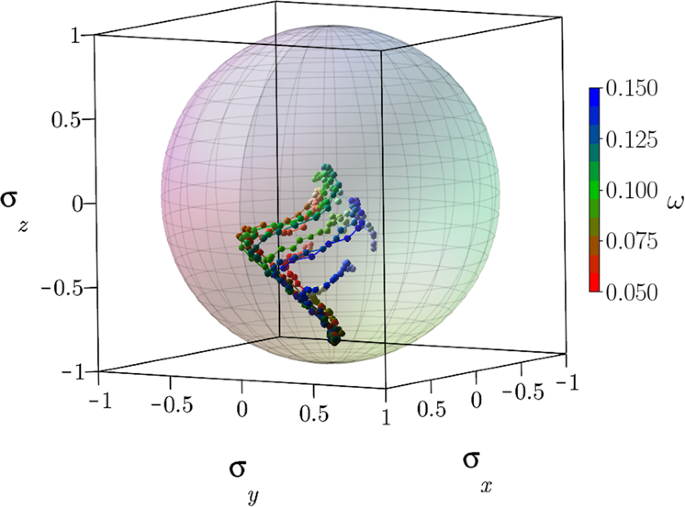Fig. 4: Single-qubit state trajectories with respect to simulated time for a range of frequencies.

### Two-qubit Hamiltonians

By identifying frequencies of interest on the qubits themselves, we can construct systems which respond uniquely to the bath, allowing for selective transitions between different eigenstates of the system. With this in mind, we first simulate a two-qubit system with a local Hamiltonian defined by:

$$H({\omega }_{1},{\omega }_{2})={\omega }_{1}{\sigma }_{z}^{1}+{\omega }_{2}{\sigma }_{z}^{2}.$$
(4)

where $${\sigma }_{z}^{j}$$ refers to the Pauli-Z matrix in Eq. (2) acting on the jth qubit. This Hamiltonian has the computational basis as its energy eigenbasis, and it can be shown that changing a frequency ωi can lead to a small energy transition between states differing locally on qubit i. By scanning over single-qubit frequencies, we can obtain a simple noise profile, and then choose appropriate ωi to influence the system. Using these frequencies, we highlight four different cases in Fig. 5a, b, c, d, showing interacting and non-interacting frequencies for each qubit. In addition, we present the calculated transition rates for each population in Table 1.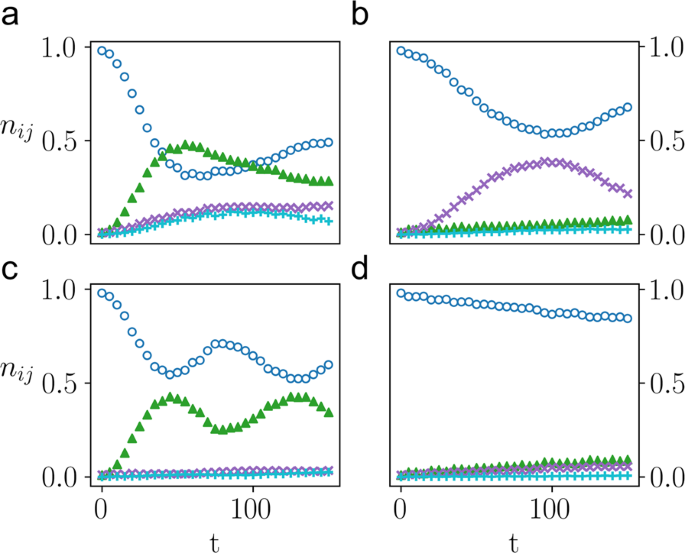Fig. 5: Simulated time evolution of a two-qubit local system which demonstrates state transitions on local sites.

The system here demonstrates asymmetry between the two qubits, with stronger coupling present on the second qubit. For large ω1 and ω2, which do not strongly couple the bath and qubit system, we witness a region of linear decay toward a uniformly depolarized state. For smaller ω1 and ω2, we clearly have a very dynamic system, allowing for transitions amongst all four states.

We can expand this idea naturally to look at a potentially non-local Hamiltonian, namely:

$$H({\omega }_{1},{\omega }_{2},{\omega }_{3})={\omega }_{1}{\sigma }_{z}^{1}+{\omega }_{2}{\sigma }_{z}^{2}+{\omega }_{3}{\sigma }_{z}^{1}{\sigma }_{z}^{2}.$$
(5)

The time propagator for this step is still relatively simple, as all elements commute and there is no Trotterization error. In addition, the eigenstates correspond to elements of the computational basis. Practically, the propagator requires only 2 CNOT gates with a sequence of exponential Z rotations. If we consider the eigenstate state with density matrix $${\rho }_{0}=\left|11\right\rangle \left\langle 11\right|$$, we can elucidate information on the eigenstates and relative differences between states. Given the Hamiltonian above, we can describe state-to-state transitions with energy gaps given by:

$${\epsilon }_{00}-{\epsilon }_{11}=2({\omega }_{1}+{\omega }_{2})$$
(6)
$${\epsilon }_{01}-{\epsilon }_{11}=2({\omega }_{1}-{\omega }_{3})$$
(7)
$${\epsilon }_{10}-{\epsilon }_{11}=2({\omega }_{2}-{\omega }_{3}).$$
(8)

For local Hamiltonians, transitions from the $$\left|11\right\rangle$$ state are predominantly induced by local transitions (ϵ00 − ϵ11 for Eq. (4) is ω1 + ω2, and so if ω1 and ω2 are small we can induce other transitions simultaneously). For this correlated Hamiltonian we can independently control energy levels, and thus have more control over the available transitions. However, we also know that noise from multi-qubit gates will be stronger, resulting in a quicker decay process. Figure 6 shows simulated evolution for two sets of frequencies demonstrating different potential behaviors which the system exhibits in response to the bath. The evolution here involves 144 time steps, or 288 CNOT gates, which can be seen to eventually lead to a fully mixed state where each population is ~1/4. In Fig. 6a, we choose frequencies that do not demonstrate a particular bath response, i.e., a large ω3, ω1, and ω2. As a result the system displays exponential decay. In Fig. 6b, we choose a large ω3 with small values of ω1 and ω2, which in principle allows for a correlated transition from the $$\left|11\right\rangle$$ eigenstate to the $$\left|00\right\rangle$$ eigenstate. Despite the presence of a stronger bath, we still see a transition between these eigenstates. When comparing the simulations in Fig. 6a and b, the $$\left|00\right\rangle$$ and $$\left|11\right\rangle$$ populations differ along the simulated trajectory by 0.13 and 0.12, respectively, whereas the $$\left|01\right\rangle$$ and $$\left|10\right\rangle$$ populations differ by only 0.028 and 0.023, respectively. Thus, we demonstrate that we can characterize the unique system-bath behavior of a correlated simulated quantum system.Fig. 6: Demonstration of a $$\left|11\right\rangle \to \left|00\right\rangle$$ eigenstate transition in the simulated time evolution of a correlated two-qubit system.

### Conclusions

By characterizing noise properties of the system, we may be able to design better error mitigation techniques or approaches to the simulation of open quantum systems where the quantum computer’s noise is harnessed as an effective bath32. For example, examining the spectral profile of the bath from the simulation of stationary quantum states may provide a unique spectroscopic fingerprint of the quantum computer. With such a fingerprint we may be able to design simulation algorithms that account for this fingerprint, providing a potentially elegant approach to error mitigation for real-world applications. Furthermore, there may be certain scenarios such as open-quantum-system simulation where the presence of noise may be a beneficial quantum resource. This idea was recently demonstrated for qubit-like spin radical systems with the decoherence of qubits being utilized33. To model an open-quantum system, we may be able to use the quantum computer’s noise to represent a significant part of the model’s bath. Use of the quantum computer’s inherent noise could potentially permit the simulation of an open quantum system at a significantly reduced computational cost in terms of both gate and qubit resources. The present approach also provides insights into controlling noise relative to a simulated timescale. Similar to extrapolation schemes for error mitigation54, we could use knowledge of the bath interaction to manipulate the noise strength as a function of the propagation step in time.

In addition, although noise sources in driven evolution cannot typically be attributed to singular sources, the absence of such specificity is not necessarily an issue for practical quantum computing. As mentioned above, characterizing whether the transverse signal is a systematic overrotation or an errant noise source is critical in calibration but not so important for system applications. For a complex quantum simulation, the effects of a single source of error (unless uniquely distinct) cannot be easily distinguished amidst the entire chorus of noise sources. From the complex set of instructions on a quantum computer emerges a complex noise profile, which is manifest in the difficulty of simulating multi-qubit noise phenomena. Through a simulated system-specific approach, we can utilize the effective bath’s spectroscopic information to design more device-specific techniques and algorithms that could improve future applications.

In this work, we simulate the time evolution of arbitrary stationary quantum states on a noisy quantum computer through the application of the time evolution operator. Noise causes a system-specific response which exhibits Markovian and non-Markovian behaviors for certain frequency domains. Spectroscopic analysis of this time evolution provides a frequency spectrum—a spectroscopic fingerprint—of the noise of the effective bath induced by the quantum computer. Understanding the noise profile may allow us to create parameterized systems in which we influence state transitions with the quantum device serving as a non-Markovian bath. The characterization of the bath is shown to be robust through simulations on multiple IBM superconducting-qubit quantum computers with different qubit numbers, connectivities, and fidelities. Although the present work employs superconducting-qubit quantum computers, in future work we plan to use this approach to characterize the noise on other types of quantum computers such as ion-trap quantum devices. These ideas provide a further step toward harnessing the unique quantum noise profile which emerges from the perspective of a simulated system on a quantum computer, that could be utilized in approaches for error mitigation and the simulation of open quantum systems.

## Methods

In each simulation we use atomic units, and the time steps are relative to meaningful scales on the quantum device. While we could associate the results to a physical time through the known gate lengths, we focus on presenting the time evolution from the perspective of the simulated system, which can have arbitrary energies and time values, and which ultimately is beholden to the gate errors. As mentioned in the text, the system Hamiltonian has a single qubit or in the two-qubit system, a sum of two-single-qubit gates, both of which can be implemented as exact exponentials. These are implemented with U3 gates on the quantum computer, which have the form:

$${U}_{3}(\theta ,\phi ,\lambda )={R}_{z}(\phi ){R}_{x}\left(-\frac{\pi }{2}\right){R}_{z}(\theta ){R}_{x}\left(\frac{\pi }{2}\right){R}_{z}(\lambda )$$
(9)
$$=\left(\begin{array}{rc}\cos \left(\frac{\theta }{2}\right)&-{{{{{{\rm{e}}}}}}}^{i\lambda }\sin \left(\frac{\theta }{2}\right)\\ {{{{{{\rm{e}}}}}}}^{i\phi }\sin \left(\frac{\theta }{2}\right)&{{{{{{\rm{e}}}}}}}^{i(\phi +\lambda )}\cos \left(\frac{\theta }{2}\right)\end{array}\right).$$
(10)

In preparing the manuscript, the user-input basis gate sequence for the ibmq devices was updated, so that now the U3 transformation is implemented as a series of 3 Rz gates interleaved with $$\sqrt{X}$$ gates (using the identity $${R}_{x}(\frac{\pi }{2})={{{{{{{{\rm{e}}}}}}}}}^{-\frac{i\pi }{4}}\sqrt{X}$$). The correlated two-qubit example requires 2 CNOT gates in addition to the U3 sequences.

Each circuit (representing a particular time point) is prepared by evolving in time according to the given time step, and then measuring the particular step 213 times. Sampling errors throughout are smaller than the depicted markers. The simulations use cloud-available quantum devices accessible through IBM Quantum Experience. The particular results reported here are performed on ibmq_armonk, ibmq_rome, ibmq_belek, ibmq_casablanca, and ibmq_bogota. The devices use fixed-frequency transmon qubits with co-planer waveguide resonators55,56. We use the Python package Qiskit (v0.17.0)57 to interface with the device. Specific device properties relevant to each run can be found in Supplementary Note II.